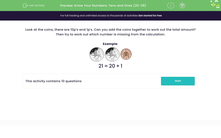# Understand Place Value Using Tens and Ones

In this worksheet, students will use tens and ones to solve money problems.Key stage:  KS 1

Curriculum topic:   Number: Number and Place Value

Curriculum subtopic:   Recognise Tens and Ones

Popular topics:   Place Value worksheets

Difficulty level:#### Worksheet Overview

In this activity, we will be adding coins.

We will be adding 10ps and 1ps.

We can think about place value to help us work these out.

Let's add up these coins together:In the tens column, we count the coins in 10s. There are 4 of these coins so we need to count in 10s 4 times:

10, 20, 30, 40

So, we have 40p in the tens column.

Now, let's count the ones column. There are 7 of these coins so we need to count in 1s 7 times:

1, 2, 3, 4, 5, 6, 7

So, we have 7p in the ones column.

Now we can just put the tens and ones together: 40 + 7 = 47p

Example:

Can you add the coins together to work out the missing number in the calculation below? Write the number in the box below.20 + ? = 21

What is missing in this number sentence?

We have the total as that number is after the equals sign.

We have the tens as these are the numbers that end in zero, there are two 10s so that makes 20.

This means we are missing the pennies. Count how many 1ps there are and that is the missing number.

There is 1p.

 20 + 1 = 21

Are you happy with that?Then let's get started!

### What is EdPlace?

We're your National Curriculum aligned online education content provider helping each child succeed in English, maths and science from year 1 to GCSE. With an EdPlace account you’ll be able to track and measure progress, helping each child achieve their best. We build confidence and attainment by personalising each child’s learning at a level that suits them.

Get started